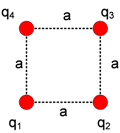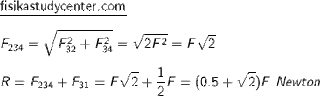# Fisika Study Center

## 4 Charges at a Square

4 charges with the same in sign are located at a square as this below figure. You have to determine the coulomb force at one of the charges, the length of square side is a meter.Here is an alternative solution for this case, you might have your own. Choose one charge, in this solution q3 is choosen. Sketch the all electrostatic forces that are acting on it cause of its interactions with the other charges. See the figure belowFind the magnitudes of each forces F32, F34 and F31The next step, you have to sum F32 and F34, of course in a vector operation, the angle is 90°. Keep in mind that kq2/a2 named F in here to simplify the calculations.Finally insert the values of F and k to get the final result. The same method and the same results can be applied for the three other charges.

Joomla Extension: by JoomlaShack
Template Upgrade by Joomla Visually